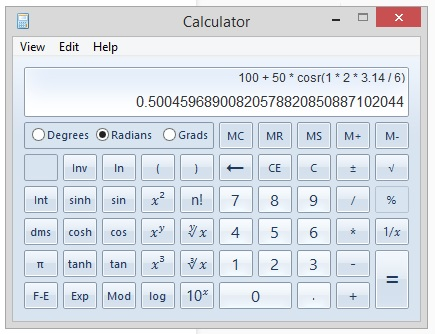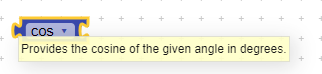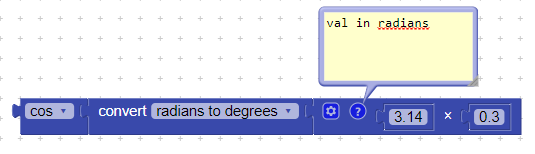# A Math problem, the result formula is wrong, why?

can everybody help me with this formula :
X1 = x + radius * cos( n * 2 * 3.14 / 6 )
when i using calculator with x = 100, radius = 50 & n = 1 the result in X1 = 125 but when i try to using kodular the result is 149.99166 this my blocks

did my formula is wrong?

Maybe you are working in radians and this wirks with degrees

1 Like

@asimjib93 as af as i known the math rule is,

1. Firstly, calculate inside the bracket or cosr(123.14/6) = 0.5,
2. Secondly, 50 * 0.5 = 25,
3. and the last is 100 + 25 = 125,
this is the basic math i knowi thought before (in kodular) i can make the math formula in a single block so it will be easier but its not, i must calculate the cos/sin outside the block i already made (i must rewrite my block).

thanks

As @pipechela has pointed out, you’re using radians in your cosine function (cosr).
But the math block takes inputs in degrees, as shown below:You should convert radians to degrees first, and then pass it to the cos block, like this:@Vishwas & @pipechela i try to rewrite as you suggested, thx

1 Like